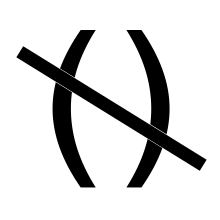I ran into the following Scala pitfall when refactoring some code recently.

## The problem

Futures sometimes execute in expected order, other times not. Testing with `Await.result(f)` didn’t block. The world was no longer deterministic. Why? Unit.

## The code

This was the code before:

``````def makeFut1():Future[Int] = Future.successful( 1 + 1)
def makeFut2():Future[String] = Future.successful( "foo" + "bar" )
def doSideEffect(a:Int,b:String):Unit = println(s"[\$a] [\$b]")

def doWork():Future[Unit] =
for {
futA <- makeFut1()
futB <- makeFut2()
} yield doSideEffect(futA,futB)
``````

Alas synchronous `doSideEffect(...)` method was refactored to be async, becoming:

``````def doSideEffect(a:Int,b:String):Unit = Future { println(s"[\$a] [\$b]") }
``````

What broke? Nothing. Scala compiled and ran it just fine. But, WFT? We’re yielding a `Future[Unit]` not a `Unit`, shouldn’t that make `doWork()` return a `Future[Future[Unit]]` and fail type checking?

In short, the Scala Spec section 6.26.1 says,

If e has some value type and the expected type is Unit, e is converted to the expected type by embedding it in the term { e; () }.

The following code would be transformed accordingly:

``````def intUnit(n:Int):Unit = n*2        // pre-compile
def intUnit(n:Int):Unit = {n*2; ()}  // post-compile
``````

This gets tricky with type parameters. If you returned `Future[Future[Unit]]`, you’re really returning `Future[Unit]`.

## Compiler Don’t Care `bout Unit

Thus, we see that by returning the Unit type, we’re really returning Void, and lose any type checking of the return type at all. As such, the compiler doesn’t give a damn1 what we return. Any `Future` executed in said yield will probably be invoked, but not as this flatmapping chain of futures, and not in the order you’d expect.

`doSideEffect(...)` is invoked, and it’s Future created, but said Future isn’t tied to this sequence of Futures. Thus, the Future returned by `doWork()` won’t wait for it, returning `Unit` immeadiately.

## Don’t Return Unit

Only use return type Unit for Void functions (a.k.a. Procedures). Using Unit to parameterize a type effectively negates type checking on that type, and loses the guarantees you’ve come to expect from the type system and compiler.

An alternative to the above example, using a sealed algebra for return state, would be:

``````sealed trait Result
object Good extends Result

def makeFut1():Future[Int] = Future.successful( 1 + 1)
def makeFut2():Future[String] = Future.successful( "foo" + "bar" )
def doSideEffectB(a:Int,b:String):Future[Result]
= Future { println(s"[\$a] [\$b]"); Good }

def doWork():Future[Result] = {
for {
futA <- makeFut1()
futB <- makeFut2()
} yield doSideEffectB(futA,futB)
}
``````

And failed to compile, as we’d hope!

``````Sample.scala:55: error: type mismatch;
found   : scala.concurrent.Future[Result]
required: Result
} yield doSideEffectB(futA,futB)
^
one error found
``````

Horay for types!

## (Appendix) Unit Type and Void

Unit is a Unit Type from Type Theory, meaning it’s a universal singleton instance referenced by `()`, the zero tuple. Every `()` in your code points to the same Unit instance. Since all Scala value types can be converted to Unit, the compiler may change them to Unit as required for return signatures to match. Any value type2 can be converted.

Let’s decompile the following functions to see what Scala does to Unit returns:

``````def intInt(n:Int):Int = n*2
def intUnit(n:Int):Unit = n*2
``````
``````  public int intInt(int);
Code:
0: iconst_2 // Load integer 2
2: imul     // Multiply ints
3: ireturn  // return product

``````
``````  public void intUnit(int);
Code:
0: iconst_2 // Load integer 2
2: imul     // Multiply ints
4: return   // Return VOID
``````

The byte code shows Java does the math in both cases, but the Unit return discards all values and returns Void.

## (Appendix) Incorrect Unit Use

Because Unit is converted from any other value type, `Unit` can be converted to `()`. That is, Unit can be converted from a type to an instance by the complier, sort of. This can lead to confusion in code.

``````def myProcedure(n:Int):Unit = {n * n; Unit}      // pre-compiled
def myProcedure(n:Int):Unit = {n * n; Unit; ()}  // post-compiled
``````

Developers may explicitly return `Unit`, but really they are returning the Unit type, not the singleton Unit reference, `()`. The reference to the actual type is being discarded.

1. Set the `-Ywarn-value-discard` compiler flag to fail builds on Value Discarding [return]
2. value type T , `scala.Nothing <: T <: scala.Any` [return]